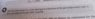# Why don't we consider ordered motion as part of the internal energy of a gas?

• Frigus

#### Frigus

Why we do not considered the translational energy of the gases particles in the internal energy due to ordered motion but only due to random motion.

Last edited:

Why we do not considered the translational energy of the gases particles in the internal energy due to ordered motion but only due to random motion.
Because we can always find an inertial frame in which the translational energy is zero; it's the center of mass frame. The internal energy is whatever cannot be transformed away just by changing frames, the energy that is there even when the center of mass is not moving.

•etotheipi, cjl, gleem and 8 others
Indeed, it's defined so by definition. All the quantities referring to the intrinsic properties of macroscopic matter are defined in the (local) rest frame of the matter, i.e., temperature, internal energy, pressure, entropy, etc. This is very important in the context of relativity theory since it tremendously simplifies things, using scalar quantities to describe the intrinsic properties of matter rather than non-covariant definitions of older treatments, which worked to some extent for special relativity but is pretty useless within the general theory.

•etotheipi and Delta2
Why we do not considered the translational energy of the gases particles in the internal energy due to ordered motion but only due to random motion.
In the general form of the first law of thermodynamics, the energy of the gas is expressed as the sum of three parts: the kinetic energy of the ordered motion KE plus the gravitational potential energy PE, plus the combined energy of random motion and molecular interaction (the internal energy U). So we write:
$$\Delta U+\Delta (KE) + \Delta (PE)=Q-W$$
So the ordered kinetic energy change is definitely accounted for.

•etotheipi, vanhees71 and Lord Jestocost
The internal energy is whatever cannot be transformed away just by changing frames, the energy that is there even when the center of mass is not moving.

Wouldn't that include potential energy in an external field (which is not part of internal energy)?

•Delta2
Wouldn't that include potential energy in an external field (which is not part of internal energy)?

OK, I didn't considered "whatever" to be limited to this thread ;)

Wouldn't that include potential energy in an external field (which is not part of internal energy)?
Sure, in non-relativistic physics it's usually customary to express everything in "quantities per mass", i.e., the energy density of an ideal fluid with velocity ##\vec{v}## may be written as
$$\epsilon=\frac{\rho}{2} \vec{v}^2 + \rho U_0(s,\rho) + \rho V(\vec{x}),$$
where ##\rho## is the mass density, ##U_0## the internal energy of the gas per unit mass as measured in its rest frame, and ##V## is the potential of the external forces per mass. E.g., for the gravitational force on Earth you may write $$V(\vec{x})=-\vec{g} \cdot \vec{x}.$$
For an ideal fluid you have no friction, heat conduction or any other dissipative transport processes by assumption, and thus the equations of motion follow under the constraint ##\delta s=0##, i.e., the entropy per mass stays constant.

•etotheipi and sophiecentaur
Um, B-level, everyone.

Why do we do not consider ordered motion as part of the internal energy of a gas?
Because wind and heat are different things.

••etotheipi, russ_watters, Frigus and 5 others
Can anyone please give the reason of the line written in the box.

#### Attachments

•IMG_20191113_093921.jpg
25.8 KB · Views: 158
It follows directly from the fact that for a non-interacting gas the equilibrium phase-space distribution is the Maxwell-Boltzmann distribution,
$$f(\vec{x},\vec{p})=\frac{\exp[\mu/(k_{\text{B}} T]}{(2 \pi \hbar)^3} \exp \left (-\frac{\vec{p}^2}{2m k_{\text{B}}T} \right).$$
From this the total number of particles and internal energy follows
$$N=V \exp[\mu/(k_{\text{B}} T)] \int_{\mathbb{R}^3} \mathrm{d}^3 p f(\vec{x},\vec{p}), \\ U=V \exp[\mu/(k_{\text{B}} T)] \int_{\mathbb{R}^3} \mathrm{d}^3 p f(\vec{x},\vec{p}) \frac{\vec{p}^2}{2m} = \frac{3 N}{2} k_{\text{B}} T.$$
For a fixed number of gas particles the internal energy only depends on temperature, not on volume. The reason is that an ideal gas is defined as particles that interact only via short-ranged interactions with the average inter-particle distance being large compared to the range of these interactions, i.e., most of the time the gas molecules are free particles and thus you can squeeze them to a certain extent without any work due to particle interactions. The only work you have to do is against the gas pressure.

Can anyone please give the reason of the line written in the box.

You mean why you reposted your initial question in photographic form? No idea.

•davenn
Because we can always find an inertial frame in which the translational energy is zero; it's the center of mass frame. The internal energy is whatever cannot be transformed away just by changing frames, the energy that is there even when the center of mass is not moving.
Thanks,
Understood today what you want to say and your reply helped me to get relief from this problem.•Delta2
Can anyone please give the reason of the line written in the box.

It is usually a very good approximation. But is doesn't work for astronomic scales.

Um, B-level, everyone.

Why do we do not consider ordered motion as part of the internal energy of a gas?
Because wind and heat are different things.
I was just seeing my old posts that I didn't understand quite well and after seeing your this answer I was just blown away because earlier in that time I was just thinking that it's answer will be out of the world but its answer is so simple that now I think that how one can even ask question like that.
Thanks.

•